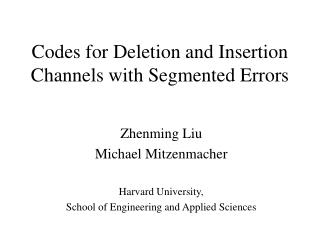Download PresentationCodes for Deletion and Insertion Channels with Segmented Errors

# Codes for Deletion and Insertion Channels with Segmented Errors - PowerPoint PPT PresentationDownload Presentation## Codes for Deletion and Insertion Channels with Segmented Errors

- - - - - - - - - - - - - - - - - - - - - - - - - - - E N D - - - - - - - - - - - - - - - - - - - - - - - - - - -
##### Presentation Transcript

1. Codes for Deletion and InsertionChannels with Segmented Errors Zhenming Liu Michael Mitzenmacher Harvard University, School of Engineering and Applied Sciences

2. The Most Basic Channels • Binary erasure channel. • Each bit is replaced by a ? with probability p. • Binary symmetric channel. • Each bit flipped with probability p. • Binary deletion channel. • Each bit deleted with probability p.

3. The Most Basic Channels • Binary erasure channel. • Each bit is replaced by a ? with probability p. • Very well understood. • Binary symmetric channel. • Each bit flipped with probability p. • Very well understood. • Binary deletion channel. • Each bit deleted with probability p. • We don’t even know the capacity!!!

4. Motivation • Capacity/coding results for deletion/insertion channels are very hard. • Very little theory for practical coding schemes. • Huge gap between codes and capacity bounds. • Perhaps this is an artifact of the model. • Are independent deletions/insertions the right model for insertions/deletions in practice? • Do different models yield much better results? • If so, would highlight challenges of original model.

5. Model Motivation • Claim: Deletion/insertion errors occur because of timing mismatches. • Mechanisms running at slightly different speeds. • Clock drift. • After one deletion (or insertion), some time passes before the next.

6. Channel Model : Segmented Deletions • Input is divided into consecutive blocks of b bits. • Channel guarantee: at most one deletion per block. • No block markers at output. • Example: b= 8. 00001110001111 0001011100101111 00010111001011 0001011100101111

7. Segmented Deletion Model • More general than models requiring a gap between deletions. • Two consecutive deletions can occur on the boundary. • Can define similar segmented insertion model.

8. Codes for Segmented Deletions :Our Approach • Create a codebook C with strings of b bits. • Codeword is concatenation of blocks from C. • Aim to decode blocks from left to right, without losing synchronization, regardless of errors. • Questions: • How can this be done? • What properties does C need? • How large can C be?

9. Notation • Let D1(u) be all strings obtainable by deleting 1 bit from u. • And • Codebook C is 1-deletion correcting if • Fixed map from strings with 1 deletion to codeword. • Our C will have this property. • Let pref(u) be first k – 1 bits of k-bit string u, and suff(u) be last k – 1 bits. • Similarly define pref(S), suff(S).

10. Intuition • At start of decoding, after reading first b – 1 bits, we know the first block. • Assuming C is 1-deletion correcting. • But don’t know if next block starts at bit b or bit b + 1 of received string. • Is marked received 0 from 1st block or 2nd? • Can’t resolve ambiguity. • Need to make sure ambiguity does not grow. • Key invariant: each successive block starts in one of two positions. Sent : Received : 00100100???????? 00100100…

11. Theorem Statement • For a segmented deletion channel with blocklength b, consider a codebook C of strings of length b satisfying: • Such a codebook allows linear time left-to-right decoding.

12. Proof Sketch • Maintain invariant: suppose block starts at position k or k + 1 of received string R. To decode block: • Done if • Otherwise • and this determines the sent block. • As long as sent block not of form • next block starts at position k + b – 1 or k + b.

13. Finding Valid Codebooks • Restrictions lead to independent set problem. • Each possible b-bit codeword is a vertex. • Throw out vertices for restricted strings. • Edge between two vertices u, v if • Maximum independent set = largest codebook. • Can be found exhaustively for small b. • Use heuristics (greedy) for larger b.

14. Results • Codes from exhaustive search: • 8 bit blocks, 12 codewords : rate > 44% • 9 bit blocks, 20 codewords : rate > 48% • Codes from heuristics: • 16 bit blocks, 740 codewords : rate > 59%. • Decoding simple – easily done in hardware.

15. Insertions • Can analyze segmented insertion channels the same way. • Surprising result: the codebooks for insertions and codebooks for deletions have the same properties! • Non-obvious symmetry!

16. Improvements • Extended scheme simulated in extended version of paper. • Ideas: • Increase C so that multiple decodings are locally possible (per block). • Use parity checks (local/global) to remove spurious decodings. • Use dynamic programming to enforce globally consistent decoding. • Results in higher rates, but slower, and currently no provable guarantees.

17. Conclusions and Open Questions • Codes ready for implementation. • Any users? • Theoretical limits. • Capacity bounds for segmented channels? • Time/capacity tradeoffs? • Possible improvements. • Analysis of more general dynamic-programming based scheme?